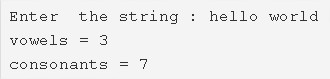# C Program Count Number Of Vowels & Consonants In A String | 4 Ways

C program to count the total number of vowels and consonants in a string – In this article, we will discuss the multiple means to count the total number of vowels and consonants in a string in C programming.

Suitable examples and sample programs have also been added so that you can understand the whole thing very clearly. The compiler has also been added with which you can execute it yourself.

The means mentioned in this piece are as follows:

• Using Standard Method
• Using Function
• Using Recursion
• Using Pointers and While Loop

A string is nothing but an array of characters. The value of a string is determined by the terminating character. Its value is considered to be 0.Firstly, you need to enter a string whose vowels and consonants you need to determine.

Here, the string “hello world” is entered.

As you can see, there are:

Vowels: 3

Consonants: 7

Hence, the numbers will be printed accordingly.

Thus, the different methods to do so in C programming are as follows:

## Using Standard Method

1. Read the entered string and store the string into the variable ‘s’ using gets(s) function.

2) For loop iterates through string ‘s’ with the structure for(i=0;s[i];i++),

a) If the ASCII value of s[i] is in the range of 65 to 90 or 97 to 122 then check s[i] is equal to any one of the vowels(a,e,i,o,u).If s[i] is equal to any vowel then increase the vowel count, otherwise increase the consonant count.

Repeat this step up to end of the string.

3) Print the number of vowels and consonants present in the string.

Output:

### Using Function – Count Total No of Vowels & Consonants

1. The main() calls the stringcount() function, passing the string as an argument to the function.

2) The function stringcount(char *s) counts the number of vowels and consonants present in the string.

a) The function checks the each character’s ASCII value is within the range of alphabets or not.

b) If the character is an alphabet then it compares the character with vowels. If the character is a vowel then increase the vowel count, otherwise increase the consonant count.

These a,b steps will be repeated up to end of the string using for loop with the structure for(i=0;s[i];i++).

3) The function stringcount(char *s) prints the vowels count and consonant count.

Output:

#### Using Recursion

1. The main() function calls the stringcount(char *s) function by passing the string as an argument.

2) The function stringcount(char *s)

initialize vowels=0,consonants=0.

a) If s[i] is null then print the vowels count and consonants count.

b) if s[i] is not null then check s[i] is an alphabet or not, based on ASCII value.

b.1) If s[i] is an alphabet then compare s[i] with vowels if it is a vowel then increase the vowel count otherwise increase the consonant count.

b.2) i value will be increased by 1. The function calls itself.

The function calls itself recursively up to s[i] is equal to null.

Output:
##### Using Pointers And While Loop
1. p is the pointer variable which points the string s.

2) While loop checks first character of the string existed or not.If it has existed then check it is an alphabet or not.

a) If it has existed then check it is alphabet or not. If it is an alphabet then check it is vowel or consonant. If it is a vowel then increase the vowel count otherwise increase the consonant count.

b) Increase the p count, then pointer variable points the next character of the string. Then check the while condition for the next character of the string.

Repeat until the character at pointer variable p becomes null.

3) Print the vowels count and consonants count.

Output:
x

## C Program To Print Number Of Days In A Month | Java Tutoring

C program to input the month number and print the number of days in that ...